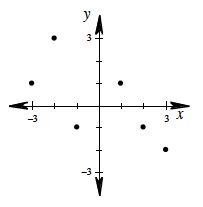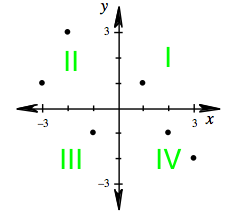### Home > AC > Chapter 1 > Lesson 1.1.2 > Problem1-14

1-14.Use the graph at right to answer the following questions about quadrants and coordinates of points.

1. What are the coordinates of the two points in Quadrant II (where the $x$-value is negative and the $y$-value is positive)?

Match the number on the $X$ coordinate with the number on the $Y$ coordinate of the two points in Quadrant II.
($−3$, $1$) and ($−2$, $3$2. What are the coordinates of the two points in Quadrant IV (where the $x$-value is positive and the $y$-value is negative)?
($2$, $−1$) and ($3$, $−2$)